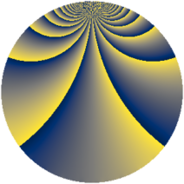Properties

 Label 3267.1.wLevel $3267$ Weight $1$ Character orbit 3267.w Rep. character $\chi_{3267}(118,\cdot)$ Character field $\Q(\zeta_{30})$ Dimension $24$ Newform subspaces $2$ Sturm bound $396$ Trace bound $1$

Related objects

Defining parameters

 Level: $$N$$ $$=$$ $$3267 = 3^{3} \cdot 11^{2}$$ Weight: $$k$$ $$=$$ $$1$$ Character orbit: $$[\chi]$$ $$=$$ 3267.w (of order $$30$$ and degree $$8$$) Character conductor: $$\operatorname{cond}(\chi)$$ $$=$$ $$99$$ Character field: $$\Q(\zeta_{30})$$ Newform subspaces: $$2$$ Sturm bound: $$396$$ Trace bound: $$1$$

Dimensions

The following table gives the dimensions of various subspaces of $$M_{1}(3267, [\chi])$$.

Total New Old
Modular forms 360 88 272
Cusp forms 72 24 48
Eisenstein series 288 64 224

The following table gives the dimensions of subspaces with specified projective image type.

$$D_n$$ $$A_4$$ $$S_4$$ $$A_5$$
Dimension 8 0 16 0

Trace form

 $$24 q - q^{4} + 3 q^{5} + O(q^{10})$$ $$24 q - q^{4} + 3 q^{5} - 4 q^{14} - q^{16} - q^{20} + 8 q^{23} + q^{31} + 6 q^{37} + 3 q^{47} - q^{49} + 2 q^{53} - 4 q^{58} - q^{59} - 6 q^{64} + 12 q^{67} - 4 q^{70} - 6 q^{71} + 2 q^{80} - 16 q^{89} - 2 q^{92} + q^{97} + O(q^{100})$$

Decomposition of $$S_{1}^{\mathrm{new}}(3267, [\chi])$$ into newform subspaces

Label Dim. $$A$$ Field Image CM RM Traces $q$-expansion
$a_{2}$ $a_{3}$ $a_{5}$ $a_{7}$
3267.1.w.a $8$ $1.630$ $$\Q(\zeta_{15})$$ $D_{3}$ $$\Q(\sqrt{-11})$$ None $$0$$ $$0$$ $$1$$ $$0$$ $$q-\zeta_{30}^{13}q^{4}-\zeta_{30}q^{5}-\zeta_{30}^{11}q^{16}+\cdots$$
3267.1.w.b $16$ $1.630$ 16.0.$$\cdots$$.1 $S_{4}$ None None $$0$$ $$0$$ $$2$$ $$0$$ $$q-\beta _{1}q^{2}+\beta _{2}q^{4}+(1+\beta _{2}-\beta _{8}-\beta _{10}+\cdots)q^{5}+\cdots$$

Decomposition of $$S_{1}^{\mathrm{old}}(3267, [\chi])$$ into lower level spaces

$$S_{1}^{\mathrm{old}}(3267, [\chi]) \cong$$ $$S_{1}^{\mathrm{new}}(1089, [\chi])$$$$^{\oplus 2}$$